Resources Section

Web Application for Symmetrical Componets: http://personal.strath.ac.uk/steven.m.blair/seq/

Do you have a question? Click on the "Questions & Answers forum" and ask away!

This video was brought to you by GeneralPAC.com, making power systems Intuitive, Open and Free for Everyone, Everywhere. Consider subscribing and supporting through patreon.com/GeneralPAC. This is a mechanism for you to support us financially so we can continue making high quality power system video tutorials. Our corporate sponsor for this topic is AllumiaX.com from Seattle, Washington. Contact them for industrial and commercial power system studies.

Principles of Symmetrical Components, Part 3C. In this part, we're going to look at how these A-operators could really help simplify our positive and sequence symmetrical component. Let's get started.

This is what we described in the previous video tutorials. We have an unbalanced set of three phasors, and the positive sequence component, negative sequence component, and zero sequence component, decomposition of the unbalanced set of phasors. So how do these a operators fit into this picture? Well, here's how they fit. Notice that the positive sequence component and negative sequence component are both balanced. That's the key term, that they are both balanced, which means for the positive sequence component, as you can see, all three phasors are displaced by 120 degrees, right? And they are equal magnitudes. That's what it means to be balanced phasors. So if we wanted to represent IC and IB with respect to IA, what do we have to do?

This is very similar to part 3A and 3B. All we would need to do is just take IA and rotate it either 120 degrees or 240 degrees to make it equal either IC or IB. All right, let's do it.

Okay, so we know that the a operator, that's gonna equal one at 120 degrees. The a superscript two operator is going to equal one at 240 degrees and the a superscript three operator, which we don't use, but it's nice to clarify, that equals one at 360 degrees. Okay, so let's get started with the positive sequence component.

So we want to represent IC with respect to IA. We would have to take IA, the positive sequence component, and then we would have to shift IA ... So we have to shift IA 120 degrees in the counterclockwise direction, which means we just multiply IA by the a operator. Simple, right? Now to represent IB, we would have to do the same thing. So we know that IB positive sequence is going to equal IA positive sequence but instead of rotating 120 degrees, now we have to actually rotate 240 degrees, which means that we're now using the A superscript two operator instead. So now it's going to be a superscript two. Pretty simple, right?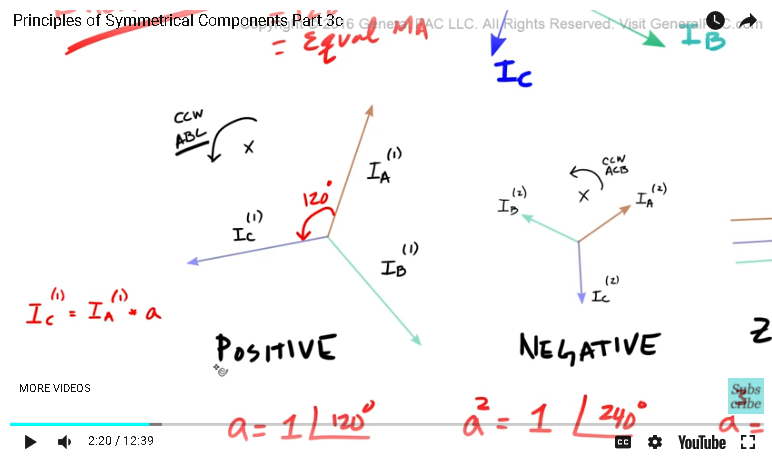And then, of course, IA positive sequence, since this is the reference phasors, we don't need to rotate it. It's already at whatever phasors it is, so we're using this as a reference. We don't need to rotate it in any direction. Now let's do the same thing with the negative sequence component. Now the negative sequence component, it's going to be a little bit different, right? But the same principles apply. What's different is that it has the same magnitudes, right? And it's displaced by the same 120 degrees, which makes it a balanced system, but it has an ACB phase sequence, that's the difference and that's the key. So if I wanted to represent IB negative sequence with respect to IA negative sequence, what would I have to do? I would have to take IA and then rotate IA by 120 degrees, which means I have to use the a operator.

Now it was different for the positive sequence. For the positive sequence, I had to rotate IA by 240 degrees, but for the negative sequence component, I only have to rotate IA by 120 degrees to make it equal IB, so that's the difference. And then, if course, IC negative sequence, that is equal to IA negative sequence, and then we have to shift IA by 240 degrees. So that is A superscript 2. Simple, right? And then, of course, IA negative sequence, that is just equal to IA negative sequence, we're not doing anything with the negative sequence. We're essentially using this as reference so we don't have to shift it.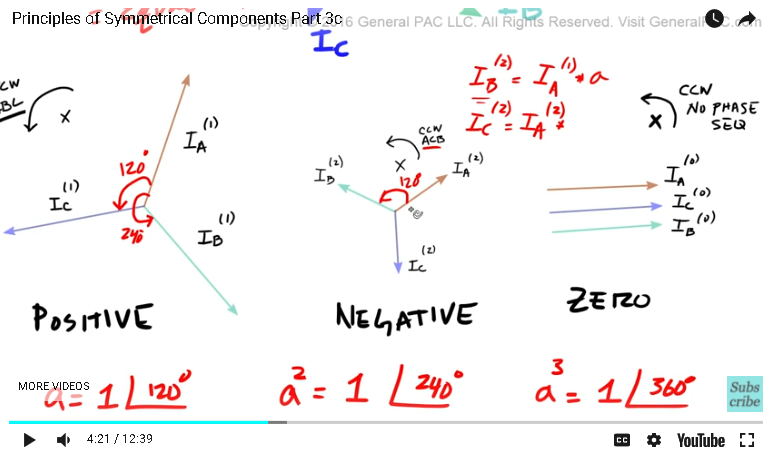Now with the zero sequence component, since this technically isn't a balanced set of phasors and that it has no phase sequence but they all have the same magnitude, we don't need to shift anything. We know that IB ... Well, we could just represent that easily by IA, we don't need to shift anything, right? And then that can also be represented by, again, we don't need to shift anything. So as you can see, the a operators make our jobs a lot easier.

Okay, so now, let's look at the benefit of the a operator. So let's backtrack just a little bit. Remember this diagram? We said that IA was equal to IA positive sequence plus IA negative sequence plus IA zero sequence and that equaled our original IA phasors. Similarly, IB positive sequence plus IB negative sequence plus IB zero sequence equaled our original phasors, and IC positive sequence plus IC negative sequence plus IC zero sequence equaled our original IC phasors, right? So let's write this out real quick.

So here is the benefit of the a operator. Right now, all we have is nine variables, three here, and three here, and three here. Nine variables that we have to keep track of all the time through our analysis of symmetrical components. What if we took the advantage of the fact that the positive and negative component sequences are balanced, right? And took advantage of the a operators to reduce the number of variables that we have to deal with? So let's see what it looks like, given that we are using the a operator.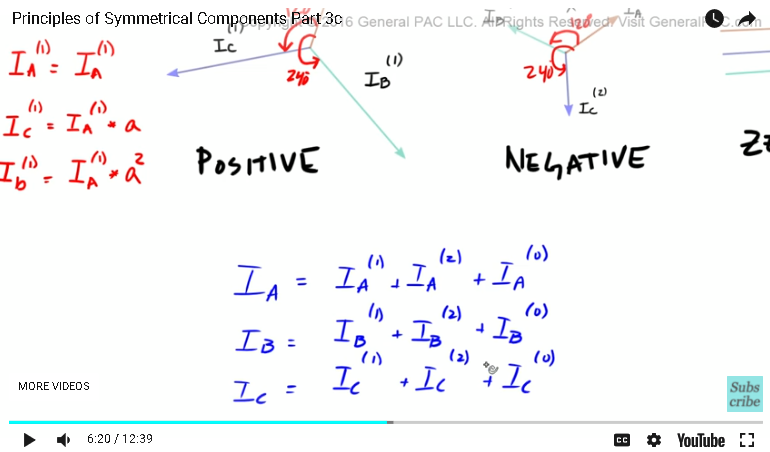Okay, so that, right there, my friends, is going to equal this. So IA ... Well, we define IA here and we said that IA was just gonna equal IA positive sequence. So we're using IA positive sequence as a reference so we don't need to shift IA positive sequence. But with IA negative sequence, which is this term here, this is how we calculated IA negative sequence. Oh, wait, IA negative sequence is also used as reference, so we don't need to shift IA negative sequence, and then IA zero sequence right there, well, we calculated that as this guy right here. So ... Which means that we don't need to shift IA negative sequence either, so that is just that.

Okay, and now let's move on to IB, and this is where the meat of the benefits are. So IB positive sequence, so let me clear this up a little bit. So IB positive sequence, which is this guy right there. Well, we calculated IB positive sequence as this guy right there. So we know that with IB positive sequence, we can just simply take IA positive sequence and rotate it by the A superscript two operator. So we can just take IA positive sequence and then we could just simply rotate it by a superscript two. Okay, I'd buy that.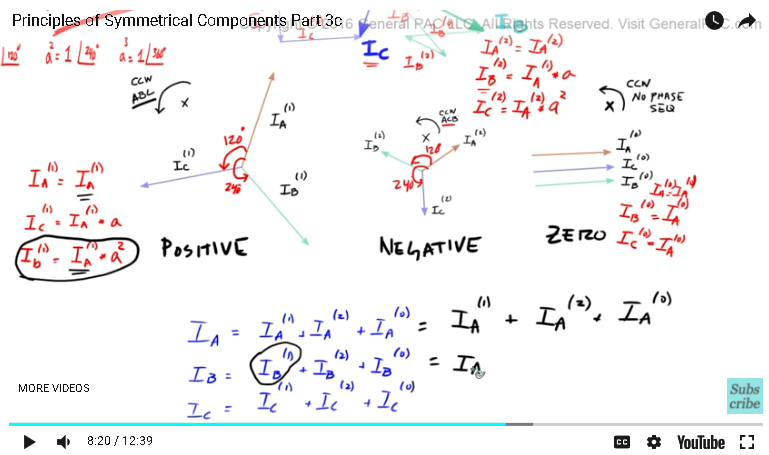Now, let's look at IB negative sequence component. So here's IB negative sequence component, right there. We said that we can take IB negative sequence component ... This should be a two right there. We said that IB negative sequence component simply equals IA negative sequence component, rotated by the a operator. So IB is equal to IA shifted by 120 degrees, right? Which means that if we just take IA negative sequence component and then rotate that by 120 degrees, that equals IB negative sequence component. And then for IB zero sequence component, since there is no shifting involved, well, that just equals IA negative sequence component, which means this equals IA negative sequence component. You see that? Let's move on to the decomposition of IC.

So we know that IC positive sequence component, right? IC positive sequence component, which is this guy right here, well, IC positive sequence component is equal to IA positive sequence component, shifted by 120 degrees, or just the a operator. So here's IC positive sequence, we're gonna take IA and then we're gonna shift it 120 degrees and that's going to equal IC positive. So we're gonna take IA and then we're gonna shift it by the a operator and that's gonna equal that. Now, let's go with IC negative sequence component, this is what we are analyzing now.

So IC negative sequence component is this guy right there, okay? Or this guy right there, so IC negative sequence component is going to equal IA rotated by 240 degrees, so that IC negative sequence component is equal to IA negative sequence component rotated by 240 degrees, right? And then IC zero sequence component, which is this guy right there, well that is simply just equal to IA zero sequence component plus IA zero sequence component. Okay, so this is getting a little bit messy but let's back up and look at what we buy with the a operators. So here, what we had before, was nine variables, nine different variables to keep track of, but using the a operators, we only had this positive sequence component. For phase a, negative sequence component for phase a and zero sequence component for phase a. So here, we only have three different variables that we need to track of. Now, granted that for each case, we are shifting one of the three variables in a particular direction but at the end of the day, we only have three variables to keep track of.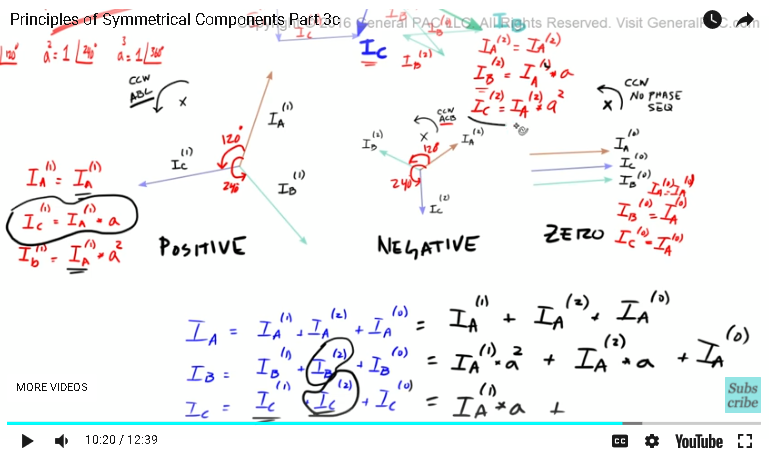So the benefit of the a operator is that we reduce the number of variables from nine to three, okay? And in the next part, we're going to represent these variables here using the matrix form. The matrix form is much easier to see and much easier to work with, and this concludes this video.

Now if you haven't subscribed already, please go ahead and click on the bottom right corner of the screen and subscribe to this channel. Visit generalpack.com for additional power system video tutorials similar to this one. And if you have any questions, there's going to be a forum link at the bottom of this video. Please go ahead and click on that link and ask away in that forum. Thank you.

Greetings from the GeneralPAC Team!

We make high-quality Power Systems Video Tutorials on complex topics that are free and open to everyone!  Thank you so much for supporting us through Patreon so can continue doing good and valuable work.

What is Patreon and why do we use it?

Patreon is a fantastic portal that allows our fans and community to make monthly contribution (like Netflix subscription) so we can continue creating high-quality power systems video tutorials. In return, you get access to incredible perks like voting on future topics, getting your questions answered, access to VIP Q/A webinars with the creators of GeneralPAC, and much more! We THANK YOU for supporting us

Why do we need your support?

An incredible amount of time and effort is needed to develop high-quality video tutorials. Each video (Part 1 for example) takes approximately 10 hours to complete which includes learning the concept ourselves, brainstorming creative ways to teach and explain the concepts, writing the script, audio recording, video recording, and editing. It's no wonder why Hundreds-of-Thousands of people have watched, liked, subscribed, and left positive comments on Youtube channel. Your support truly makes all the difference.